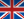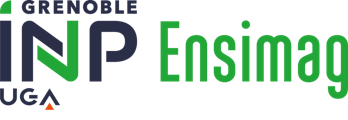Une voie, plusieurs choix
Informatique et Mathématiques appliquées# Statistical Analysis and document mining - 4MMSADM

A+Augmenter la taille du texteA-Réduire la taille du texteImprimer le documentEnvoyer cette page par mail cet article Facebook Twitter Linked In
• #### Number of hours

• Lectures : 16.5
• Tutorials : 7.5
• Laboratory works : 9.0
• Projects : -
• Internship : -
• Written tests : -
ECTS : 3.0
• Officials : Olivier GAUDOIN

### Goals

The aim of this course is to present the statistical approaches for analysing multivariate data. The information age has resulted in masses of multivariate data in many different field: finance, marketing, economy, biology, environmental sciences,...The theoretical and practical aspects of multivariate data analysis are given equal importance. This balance is achieved through practicals involving actual data analysis using the R software.

Content

1. Multiple linear regression. Least squares, Gaussian linear model, test of linear hypotheses, one-way analysis of variance.
2. Principal Components Analysis (PCA).
3. Classification, linear discriminant analysis, perceptron, Naive Bayes
4. Text mining, numeric representation of texts, connexion with graph clustering.

Prerequisites

Elementary notions in probability theory (probability distribution, joint probability density function for random vectors, conditional distribution, expectation, variance, covariance, Gaussian distribution)

Elementary notions in mathematical statistics (estimator, confidence interval, statistical tests). As a bonus: simple linear regression.

Notions in linear algebra (matrix reductions).

As a bonus: elementary notions in Rstudio and the R software.

Tests

Practical exam with R (3 h) and reports on supervised practicals.
Authorized document and material: handwritten notes only. An electronic version of the booklet will be provided by the teacher.

N1=1/2E1+1/2P
N2=E2

The exam is given in english onlyCalendar

The course exists in the following branches:

• Curriculum - Financial Engineering - Semester 8 (this course is given in english only)
• Curriculum - Information Systems Engineering - Semester 8 (this course is given in english only)
• Curriculum - Math. Modelling, Image & Simulation - Semester 8 (this course is given in english only)
see the course schedule for 2020-2021

Course language(s):You can find this course among all other courses.

Bibliography

CM BISHOP (2006) Pattern recognition and machine Learning. Springer
http://research.microsoft.com/en-us/um/people/cmbishop/prml/

C. CHATFIELD and AJ COLLINS (1980) Introduction to multivariate analysis. Science paperbacks

T HASTIE, R TIBSHIRANI, and J FRIEDMAN (2009). The Elements of Statistical Learning, 2d ed, Springer. http://www-stat.stanford.edu/~tibs/ElemStatLearn/

G. SAPORTA : Probabilités, statistique et analyse des données, Technip, 2006.

A+Augmenter la taille du texteA-Réduire la taille du texteImprimer le documentEnvoyer cette page par mail cet article Facebook Twitter Linked In

Date of update December 6, 2017École nationale supérieure d'informatique et de mathématiques appliquées
681, rue de la passerelle - Domaine universitaire - BP 72
38402 SAINT MARTIN D'HERES